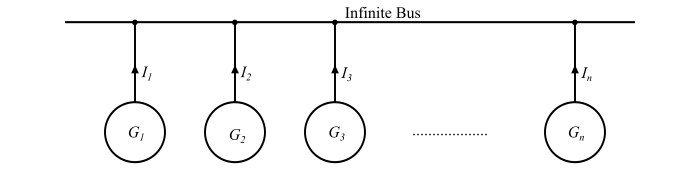# Operation of Synchronous Generator with an Infinite Bus

## What is an Infinite Bus?

Normally, in a power system, more than one synchronous generators or alternators operate in parallel. The alternators may be located at different places.

• A group of alternators located at a single place may be treated as a single large alternator.

• Also, the alternators connected to the same busbar but separated by transmission lines of low reactance may be considered as a single large machine.

The capacity of this power system is so large that its terminal voltage and frequency may be taken constant. The connection or disconnection of a single alternator or a single small load would not affect the magnitude and phase of the voltage and frequency. Therefore, the power system behaves like a large alternator having virtually zero internal impedance and infinite rotational inertia. Such kind of system having constant voltage and constant frequency regardless of the load is known as infinite busbar system or infinite bus. An infinite bus can be defined as follows −

An infinite bus is a power system so large that its voltage and frequency remain constant regardless of how much real power and reactive power is drawn from or supplied to it.

When an alternator is operating in parallel with an infinite bus and its excitation voltage is changed, the power factor of the machine changes. However, the change in excitation does not change the terminal voltage which held constant in the system.

## Characteristics of an Infinite Bus

The characteristics of an infinite bus are given below −

• The frequency of the infinite bus remains constant since the rotational inertia is too large to enable the incoming machine to alter the speed of the system.

• The terminal voltage of the infinite bus remains constant, because the incoming machine is very small to increase or decrease it.

• The synchronous impedance of an infinite bus is very small because the system consists of a large number of alternators connected in parallel.

## Operating Characteristics of an Alternator Connected to an Infinite Bus

An alternator connected to an infinite bus has the following operating characteristics −

• The terminal voltage and frequency of the alternator are controlled by the power system to which it is connected.

• The excitation of the alternator controls the reactive power supplied by the alternator to the infinite bus. The increase in the excitation of the alternator increases the reactive power output of the alternator.

• The real power supplied by the alternator to the infinite bus is controlled by the governor set points of the alternator.

## Obtaining an Infinite Bus

The figure shows n alternators connected to an infinite bus.## Proof of voltage remaining constant for an infinite bus

Let

• 𝑉 = Terminal voltage of the busbar

• 𝐸 = Induced EMF of each alternator

• 𝑍𝑠 = Synchronous impedance of each alternator

• 𝑛 = Number of alternators in parallel

Thus, the voltage equation of the system would be

$$\mathrm{𝑉 = 𝐸 − 𝐼𝑍_{𝑠\:(𝑒𝑞)} … (1)}$$

Where,

$$\mathrm{𝑍_{𝑠\:(𝑒𝑞)} =\frac{𝑍_{𝑠}}{𝑛}… (2)}$$

From eqn. (2), when n is very large, then

$$\mathrm{𝑍_{𝑠\:(𝑒𝑞)}→0;\:\:\:and\:\:\:∴𝐼𝑍_{𝑠\:(𝑒𝑞)}→0}$$

$$\mathrm{∴\:𝑉 = 𝐸\:(Cosntant) … (3)}$$

## Proof of frequency remaining constant for an infinite bus

Let

$$\mathrm{𝐽 = moment\:of\:inertia\:of\:each \:alternator}$$

Then, the total moment of inertia of all n alternator operating in parallel is

$$\mathrm{\sum\:𝐽 = 𝐽 + 𝐽 + ⋯ + 𝐽 (𝑛\:times) = 𝑛𝐽 … (4)}$$

$$\mathrm{∴\:Acceleration\:of \:alternator =\frac{accelerating\:torque}{moment\:of \:inertia}}$$

$$\mathrm{\Rightarrow\:Acceleration\:of \:alternator =\frac{τ𝑎}{\sum 𝐽}=\frac{τ𝑎}{𝑛𝐽}… (5)}$$

From eqn. (5), if n is very large, then nJ is very large, thus

$$\mathrm{Acceleration\:of\:alternator\:→\:0}$$

Hence, the speed of the machine is constant. Consequently, the frequency is constant.

Therefore, in order to obtain a constant voltage and constant frequency of a practical busbar system, i.e., an infinite busbar, the number of alternators connected in parallel should be as large as possible.